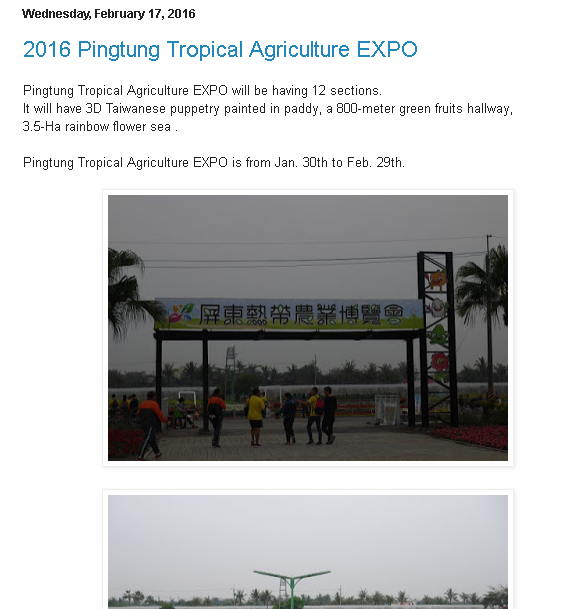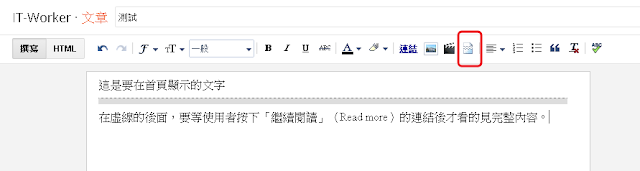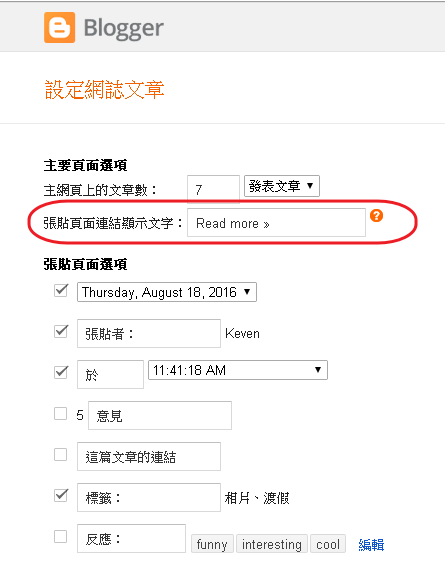```<!-- Auto Read More Body Start -->
<b:if cond='data:blog.url == data:blog.homepageUrl'>
<b:if cond='data:blog.pageType == &quot;index&quot;'>
<div expr:id='&quot;summary&quot; + data:post.id'><data:post.body/></div>
<script type='text/javascript'>createSummaryAndThumb(&quot;summary<data:post.id/>&quot;,&quot;<data:post.url/>&quot;,&quot;<data:post.title/>&quot;);</script>
</b:if></b:if>
<b:if cond='data:blog.pageType == &quot;item&quot;'><data:post.body/></b:if>
<b:if cond='data:blog.pageType == &quot;static_page&quot;'><data:post.body/></b:if>
<div style='clear: both;'/> <!-- clear for photos floats -->
<!-- Auto Read More in Body End -->
```

```<!-- Auto Read More Body Script Start -->
<script type='text/javascript'>
post_no_pic_sum = 300;
post_pic_sum = 200;
pic_h = 220;
pic_w = 300;
</script>
<script type='text/javascript'>
//<![CDATA[
function removeHtmlTag(t,e){if(-1!=t.indexOf("<")){for(var n=t.split("<"),i=0;i<n.length;i++)-1!=n[i].indexOf(">")&&(n[i]=n[i].substring(n[i].indexOf(">")+1,n[i].length));t=n.join("")}for(e=e<t.length-1?e:t.length-2;" "!=t.charAt(e-1)&&-1!=t.indexOf(" ",e);)e++;return t=t.substring(0,e-1),t+"..."}function createSummaryAndThumb(t,e,n){var i=document.getElementById(t),m="",r=i.getElementsByTagName("img"),s=post_no_pic_sum;r.length>=1&&(m='<span class="posts-thumb" style="float:left; margin-right: 10px;"><a href="'+e+'" title="'+n+'"><img src="'+r.src+'" width="'+pic_w+'px" height="'+pic_h+'px" /></a></span>',s=post_pic_sum);var g=m+"<div>"+removeHtmlTag(i.innerHTML,s)+"</div>";i.innerHTML=g}
//]]>
</script>
<b:if cond='data:blog.pageType != &quot;static_page&quot;'>
<b:if cond='data:blog.pageType != &quot;item&quot;'>
<style type='text/css'>
.post-footer {display: none;}
</style>
</b:if>
</b:if>
<!-- Auto Read More Body Script End -->
```

post_no_pic_sum = 300;  –> 這裡是指文章內沒有夾帶圖片時要顯示的字數
post_pic_sum = 200;  -> 這裡是指文章內有夾帶圖片時要顯示的字數
pic_h = 220;  -> 圖片的高度
pic_w = 300;  -> 圖片的寬度1、開啟「版面配置」
2、在「Blog Posts」的區塊上，點選「編輯」